# 3.2、抽象一个类和实例化对象PHP 同时被 3 个专栏收录19 篇文章 0 订阅

1、类的声明

【修饰类的关键字】class 类名{

}

<?php
class Base {
public function sayHello() {
echo 'Hello ';
}
}


2、类的成员属性

eg：

<?php

class test
{
public $var1 = 1; protected$var2 = 2;
private $var3 = 3; static$var4 = 4;
}


3、类的成员方法

eg:

<?php
class MyClass
{
private $foo = FALSE; public function __construct() {$this->$foo = TRUE; echo($this->$foo); } }  二、实例化对象 我们在创建类之后，并不是直接使用类，而是通过类来实例化出对象。 1、实例化对象 实例化对象需要使用的new关键字，一个类可以被实例化出多个对象，对象之间没有关联。 eg: <?php class MyClass { private$foo = FALSE;

public function __construct()
{
$this->foo = TRUE; echo($this->foo);
}
}

$bar = new MyClass();  2、对象类型在内存中分配情况 内存大体上被分为四段： 1）栈空间段 该空间段主要特点是：空间小，被访问速度快，后进先出（先进后出）。用于存储占用空间不变且占用空间小的数据类型的内存段。例如整型1、10、10000在内存中占用的空间是等长的，都是32位4字节。整型、double、boolean等类型都可以存储在栈空间段。 2）堆空间段 用于存储数据长度不定长，而且占用空间很大的数据类型的数据，例如字符串、数组、对象。该段是动态分配的。 3）初始化数据段 用于存放可执行文件中已初始化的全局变量，数据段存储经过初始化的全局和静态变量 4）代码段 存放可执行文件的操作指令，就是可执行程序在内存中的镜像。代码段需要防止在运行时被非法修改，所以只允许读取操作，不允许写入（修改）操作。例如程序中的函数就存储在该内存段中。 其各段特点简要说明如下图：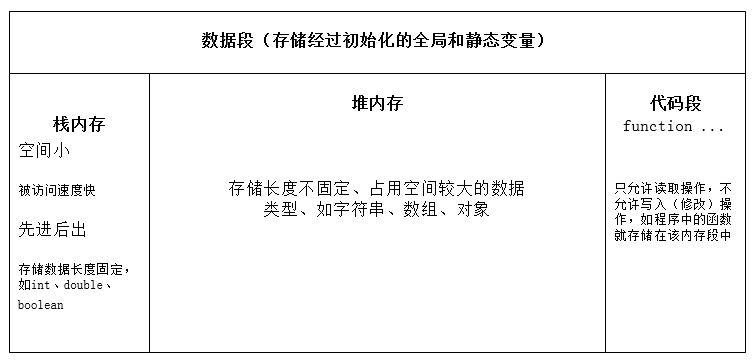实例说明：eg: <?php class person{ public$name;
public $sex; public function say(){ echo '666'; } }$p1 = new person();
$p2 = new person();$p3 = new person();
/**$p1是第一个对象的引用变量（通过“=”把第一个对象的首地址"0xff001"，赋值给了$p1），
* 存放的是一个十六进制的整数被存放在栈内存中。相当于一个指针指向堆里边的对象。
* 所以访问对象里边的成员都需要通过引用变量$p1 */  上段代码图形说明如下：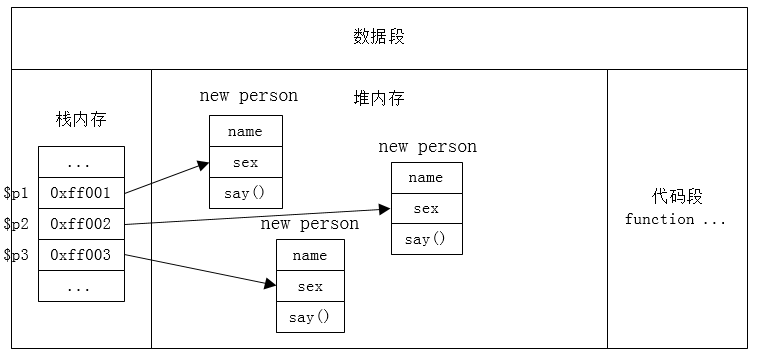易混淆点： 1、当将对象赋值给另一个变量时，此时是将对象的“首地址”赋值给了另一个变量，堆内的对象并没有复制一份。 实例说明eg1: <?php class test{ public$name;
}
$a = new test();$b = $a;//这里与$b = &$a效果是一样的，仅针对对象$b->name = 123;
var_dump($a->name);//输出123，说明只是将第一个对象的“首地址”赋值给了$b,改变的都是一个堆内的对象属性值
unset($a); var_dump($b->name);//输出123，unset释放的只是第一个对象栈中的引用变量。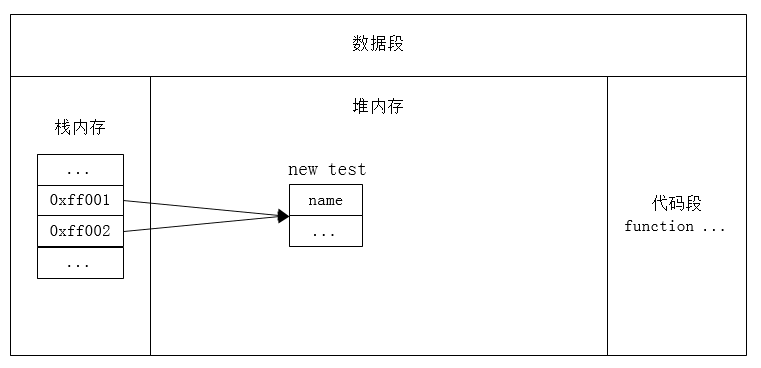2、如果想得到一个对象的副本，用$a =clone$b; 用了clone后会产生一个新对象，分配内存，独立于原来的$a。 eg2: <?php class test{ public$name;
}
$a = new test();$b = clone $a;$b->name = 123;
var_dump($a->name);//输出null unset($a);
var_dump($b->name);//输出123  示例2图形说明：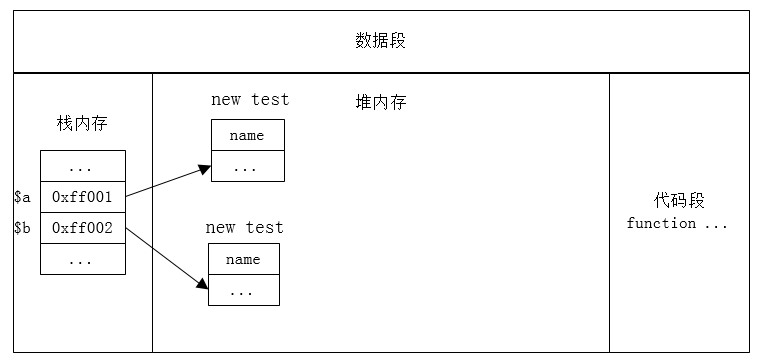3、当一个对象的引用断了的时候，这个对象在堆中就被当做垃圾，php就会执行垃圾回收机制，将这个对象回收，如图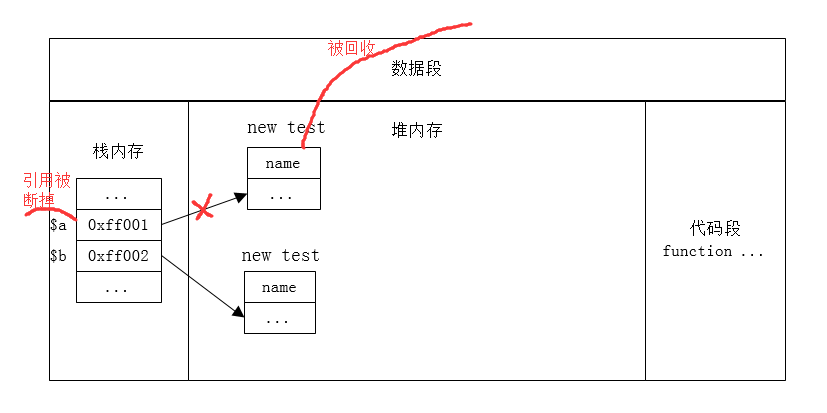4、栈内后进先出（先进后出）机制 eg3: <?php class test { public$name;
//构造函数，当类被实例化时，会自动执行
public function __construct($name) {$this -> name = $name; echo$this->name."这里被执行了<br>";
}
public function __destruct()
{
echo $this->name."我被回收了<br>"; } }$p1 = new test('王二');
$p1 = 1;$p2 = new test("张三");
$p3 = new test("李四"); /**输出 * 王二这里被执行了 * 王二我被回收了 * 张三这里被执行了 * 李四这里被执行了 * 李四我被回收了 * 张三我被回收了 */ //因为$p1引用被重新赋值，最先失去了引用，所以调用了析构函数。
//然后在这个脚本执行完成后，开始自动调调用后边对象的析构函数，
//因为$p2,$p3引用存储在栈内，因为栈内后进先出机制，最后创建的对象最先被释放，所以$p3先被销毁。  三、$this

1、构造函数__construct ([ mixed $args [,$... ]] )

eg1:

<?php
class Base {
function __construct() {
print "1";
}
}

class Sub extends Base {
function __construct() {
parent::__construct();
print "2";
}
}

class OtherSub1 extends Base {
function __construct() {
print "2";
}
}

class OtherSub2 extends Base {

}

$obj = new Base();//输出1$obj = new Sub();//输出12
$obj = new OtherSub1();//输出2$obj = new OtherSub2();//输出1


eg:

<?php
class Base {
public $name; public function __construct($name = '小王') {
$this->name =$name;
}

public function name(){
echo $this->name; } }$name1 = new Base('小李');
$name1->name();//输出‘小李’$name2 = new Base();
\$name2->name();//输出‘小王’


2、析构函数__destruct ( void )

05-062634
05-1815万+02-075800
05-03701
05-181万+
01-241205
10-27781
11-01241
07-112007
09-29126
10-22127
07-10314
03-2142
07-3137
11-2031点击重新获取扫码支付余额充值